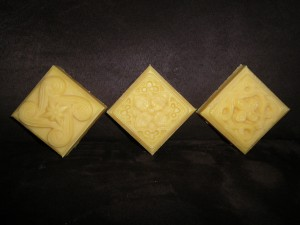In my previous post I discussed an important difference between superfat and lye discount. You might want to read it first, as I explain there why in hot process (HP) and only in hot process, the calculation of the superfat should be based on the saponification values and not on the percentage of oils.

I introduced there a formula on how to calculate the % of superfat given a recipe in the hot process method. In this post I show the other problem – How to calculate the amount of oil in order to get the desired % of the hot process superfat.

First, I repeat the first calculation:

### How to calculate the HP superfat given the amount of oils added at the end

To summarize, it is 1-B/A, where

A – is the amount of lye needed to saponify all the oils in the recipe (including those added after the soap is finished), at 0% lye discount

B – is the lye needed to saponify only the oils in the basic recipe (without superfat oils)

But what if I do want to calculate it vice-versa? I do not want to find a superfat % given the amount of my superfat oil, but I want to find how much of the oil should I add in order to get a certain superfat %!

### How to calculate the amount of oil in order to get the desired % of superfat in HP

I will use a basic recipe from a post on talksoapforum, where this question was asked by Heavensgrace.

Basic recipe:

135 g (4.8 oz) palm oil
135 g (4.8 oz) coconut oil
317 g (11.2 oz) olive oil

lye needed to saponify at 0% superfat is 87g (3.078 oz)

We want to superfat this basic recipe with shea butter and castor oil at 10%.

Question is: How much of shea butter and castor oil we need to add in order to obtain 10% superfat?

If we want a 10% superfat, we are adding oils representing 10% of the lye needed to saponify all the oils (basic recipe + superfat oils). This means the basic recipe represents a fraction fully saponified by 90% of the lye and the 100% lye needed for the recipe is calculated as

87/0.9 = 96.7g  (or 3.078/0.9=3.42 oz)  – This is the amount of lye needed to saponify 100% of oils.

Therefore we need the quantity of oils that would be saponified by 96.7g87g = 9.67g  (or 3.42-3.078 = 0.342 oz) of lye (10% of the whole lye amount). Both castor oil and shea butter have both 0.128 SAP.

The quantity of oil to get the 10% superfat would be 0.128 SAP * x = 9.67 oz => 75.5 g
(or 0.128 SAP * x = 0.342 oz => 2.67 oz) of shea butter, or 75.5g (or 2.67 oz) of castor oil, or basically any combination of the two as soon as they add up to 75.5g (or 2.67 oz) (we can do this, because they have the same saponification value)

So, to make a formula out of it:

B – is the lye needed to saponify at 0% the basic recipe (in your case it is 3.078 oz)
C – is the superfat % you wish (10%, please use 0.1)
SAP – is the saponification value of the superfat oil you want to add (0.128 for castor oil)
D – is the amount of the superfat oil we need to add to achieve C

E – is the amount of lye needed to saponify all the oils (the basic recipe + superfat oils) at 0% (in other words if superfat oil was part of the recipe and you did not superfat at all)

E = B/(1-C)
D = (E-B)/SAP

(in our example

E = 3.078/(1-0.1) = 3.078/0.9 = 3.42 oz
D = (3.42-3.078)/0.128 = 2.67 oz

)